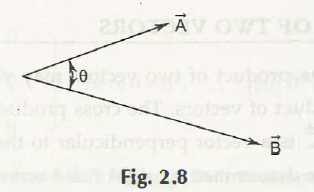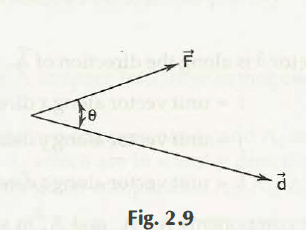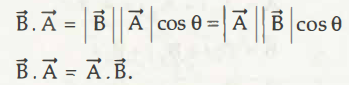The dot product is also known as scalar product of any two vectors which is a number that deals with scalar quantity and is gained just by performing any specific operation done on any vector components. The dot product comes with a meaning that is referred for pairs of vectors which may have same number of dimensions.The symbol for any dot product is represented through a heavy dot (.)In two dimensional vectors would be expressed in different terms of X coordinates and y coordinates of end points which may assume as (x, y) = (0, 0)The dot product of any two vectors may be determined just by multiplying X-coordinates and then multiplying by y-coordinates and then finally add up two products.

In case of polar coordinates, vectors can be expressed in terms of magnitude or length and direction. In such a situation, the dot product of vectors appears to be equal to that of product of lengths which is multiplied by cos θ of any angle found between them.

Links of Next Mechanical Engineering Topics:-### Customer Reviews

My Homework Help
Rated 5.0 out of 5 based on 510 customer reviews at